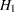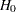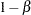The POWER Procedure
 Overview of Power Concepts

In statistical hypothesis testing, you typically express the belief that some effect exists in a population by specifying an alternative hypothesis. You state a null hypothesisas the assertion that the effect does not exist and attempt to gather evidence to rejectin favor of. Evidence is gathered in the form of sample data, and a statistical test is used to assess. Ifis rejected but there really is no effect, this is called a Type I error. The probability of a Type I error is usually designated "alpha" or, and statistical tests are designed to ensure thatis suitably small (for example, less than 0.05).

If there really is an effect in the population butis not rejected in the statistical test, then a Type II error has been made. The probability of a Type II error is usually designated "beta" or. The probabilityof avoiding a Type II error—that is, correctly rejectingand achieving statistical significance—is called the power. ( Note:Another more general definition of power is the probability of rejectingfor any given set of circumstances, even those corresponding tobeing true. The POWER procedure uses this more general definition.)

An important goal in study planning is to ensure an acceptably high level of power. Sample size plays a prominent role in power computations because the focus is often on determining a sufficient sample size to achieve a certain power, or assessing the power for a range of different sample sizes.

Some of the analyses in the POWER procedure focus on precision rather than power. An analysis of confidence interval precision is analogous to a traditional power analysis, with "CI Half-Width" taking the place of effect size and "Prob(Width)" taking the place of power. The CI Half-Width is the margin of error associated with the confidence interval, the distance between the point estimate and an endpoint. The Prob(Width) is the probability of obtaining a confidence interval with at most a target half-width.Previous Page | Next Page | Top of Page